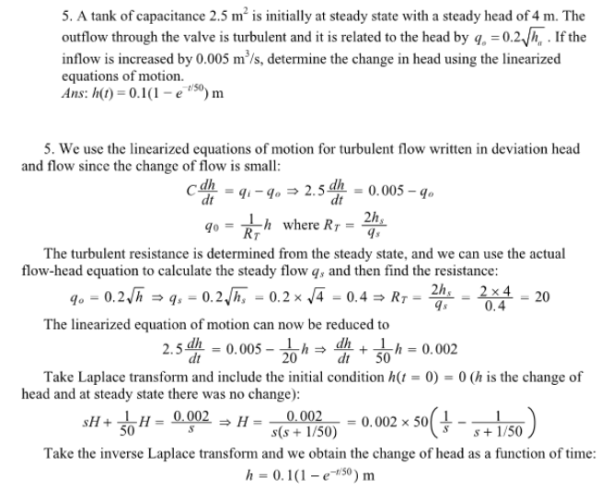A tank of capacitance 2.5 m^2 is initially at steady state with a steady head of 4 m. The outflow through the valve is turbulent and it is related to the head by q=0.2h^1/2. If the inflow is increased by 0.005 m^3/s, determine the change in head using the linearized equations of motion.A tank of capacitance 2.5 m^2 is initially at steady state with a steady head of 4 m. The outflow through the valve is turbulent and it is related to the head by q=0.2h^1/2. If the inflow is increased by 0.005 m^3/s, determine the change in head using the linearized equations of motion.

System Dynamics Page 2 dynamics dynamics dynamics dynamics dynamics dynamics dynamics System dynamics Page 3